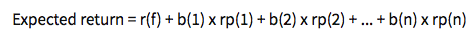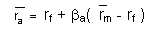# Asset Valuation – How to value an asset through their financials

Every asset has a value that is always changing. So, what factors affect this change? Things such as earnings announcements, financial ratios, and recent news all go into the movement of an asset’s valuation. The key with all asset valuation is that ratios and prices are always relative. This means most methods for asset valuation can only compare one company to another company (usually in the same industry) to give an idea of which one has a competitive edge, and is poised for growth.

The most common methods of asset valuation are based on ratios from companies’ financial statements, and some more advanced theories that try to make a more “objective” valuation, regardless of industry.

## Valuation Through Financial Ratios

The two most common ways to give relative value to companies is by comparing their Price over Earnings, and Price over Sales ratios.

### Price over Earnings (PE Ratio)

Price over Earnings = (Current Stock Price/Earnings over last 12 months)

Price to earnings and price to sales are a good place to start. For the purpose of this article, we will be looking at two different companies and their financial ratios, Nordstrom ( JWN) and Macy’s ( M). Calculate this ratio by taking the current stock price and dividing it by the company’s earnings per share (obtained from the Income Statement, which you can find under “Financials” in our quotes tool).

 JWN M Stock Price 47.71 23.5 Earnings 2.02 1.99 P/E Ratio 23.61 11.81From this point, we can interpret the value of a stock use this ratio to determine if it is a high growth or flawed stock. First we take a look at Nordstrom and how to interpret its P/E ratio. Their current P/E ratio is 23.61. Next we look at Macy’s, with a P/E ratio of 11.81. High P/E ratios correlated with higher growth stock due to investors finding more value in a companies share price. If this holds true, Nordstrom is seen as a better buy than Macy’s because investors expect more growth in the future.

### Price over Sales

Price over Sales = (Current Market Cap/Revenue over the past 12 months)

A second metric to look at is the price to sales, which while similar to price to earnings, looks to the company’s retail performance. Calculate this by taking the company’s market cap and dividing it by the revenue for the year. You can find a company’s current Market Cap on the standard Quotes page, and their revenue from their Income Statement (in the Financials section of the quotes tool, or under “Company”, and “Key Ratios”).

 2016 JWN M Market Cap (In Billions) 7.92 7.19 Revenue (In Billions) 14.86 25.35 P/S Ratio 0.530 0.280This specific financial ratio has been very useful over the past year with regards to the slump in retail stores due to online shopping. Nordstrom has a P/S ratio of 0.530 and Macy’s has a P/S ratio of 0.280. This is a great tool for valuing an asset in comparison to another in terms of sales. This ratio shows that Nordstrom’s current market cap is much lower than it could be in terms of their revenue compared to Macy’s – per dollar value of the company, Macy’s is making more sales.

### Growth of Revenue

It is also important to look at growth of revenue over the past year to determine if this ratio is useful to value. You can also find this information in the Quotes tool, under the “Company” section, and “Key Ratios”.

 JWN M Revenue Growth (3 year) 4.62% -2.78% Revenue Growth (5 year) 5.47% -0.99%

When we put all three of these together, we can get a picture of which stock is looking for more growth. The P/S and P/E ratios are both much higher than Macy’s, which means investors are expecting to see much more growth. This is backed up by the revenue over the last few years, where Nordstrom has been growing much faster, giving more value to their shareholders.

## Theories and Models:

Financial ratios themselves are a great indicator of future performance of an asset, however, there are theories and models to use to determine things such as expected return. The most pertinent ones are the arbitrage pricing theory and the capital asset pricing model, or CAPM for short. Both of these formulas use similar variables, so it is important to identify them first:

Risk-free rate (rf)

The expected return on a riskless asset such as a U.S. Treasury Bill. This is because the U.S. Treasure will never default

Risk on the Market (rm)

This is a broad risk, which includes risks faced by financial markets, but some of them include, recessions, political turmoil, changes in interest rates, etc. There are many different ways to calculate this from different sources.

Market Risk Premium (rm – rf) = rp

This is simply the risk on the market subtracted by the risk-free rate. How much an investor stands to gain by investing in a riskier asset.

Beta (β)

The measurement of the riskiness of an asset. You can get this from the Quotes page for almost any stock.

Sensitivity of an asset to a factor (b)

This is how an assets price is affected by the movement of another asset. This sensitivity can be anything – basically any variable you want to include in your model that you think will impact the price of the stock.

### Arbitrage Pricing Theory (APT)

We’ll start with the arbitrage pricing theory the formula is as follows:To use this model, start by taking the risk-free rate of return, then adding in how you think many different variables will impact the price. Each “b” in the formula is another factor you think will have an impact, and you can have as many factors as you want. You could base the formula off of the inflation rate, exchange rates, production rates, etc. The possibilities are endless.

The valuation that you attain from this formula is considered to be the expected return on an asset. Say you are trying to find the expected return on Tesla ( TSLA). A few different variables to use would be oil prices, electric prices, and auto loan rates. These three have a direct correlation with the performance of Tesla’s sales over the next year and therefore their stock price. If auto loan rates go up, you can expect Tesla’s expected return to go down. If oil prices go up you can expect their expected return to rise due to more people switching to electric vehicles. It’s a great tool to bring in variables that are otherwise disregarded.

#### Using Arbitrage Pricing Theory

To put this formula in action, first identify several factors that should impact the stock’s price, which would be the “b” factors. For example with Tesla, we might say:

• b(1) = Growth in the price of oil
• b(2) = Growth in the price of electricity
• b(3) = Growth in auto loan interest rates

Next, think about how each of these factors influence the stock price (rp, or “Risk Premium”). You can use Linear Regression to calculate these variables in Excel or any other spreadsheet program, the values below are just an example.

• rp(1) = 0.7, meaning we expect a 1% increase in the price of oil to make Telsa’s stock price to go up by 4%
• rp(2) = -0.5, meaning we expect a 1% increase in the price of electricity to make Telsa’s stock price to go down by 0.5%
• rp(3) = -2, meaning we expect a 1% increase in auto loan interest rates to make Tesla’s stock price to go down by 2%

Next, we just need to get the actual values for how we expect all of these rates to move in the next year, then plug these numbers into the formula.

• Oil prices are expected to go down in 2017 by about 4%
• Electricity prices are expected to go down in 2017 by about 2%
• Car loan interest rates are expected to go down by 3%
• The current risk-free rate (as valued by a 10 year treasury bill) is 2.35%

Finally, plug these values into the formula:

Expected Rate of Return = (rf) + b(1) * rp(1) + b(2) * rp(2) + b(3) * rp(3)

= 2.35% + (-4% * 0.7) + (-2% * 0.5) + (-3% * -2)

= 2.35% – 2.8% + 1% + 6%

= 6.55%

So, a stock has this expected return given sensitivity towards these factors of 6.55%

### Capital Asset Pricing Model (CAPM)

The capital asset pricing model is the following formula:In plain English, this formula means that our expected rate of return is based on the risk free rate, plus the Beta of our stock, modified by the risk in the overall market.

For this we simply plug in the above variables, remembering the order of operations and you will receive an expected return on an asset. The great thing about this formula is that it’s rather simple and all of the information needed is listed publicly and is easy to access. It’s important to keep in mind that even though this gives you an expected return it isn’t the most accurate number and should always have more research done about an asset before investing. We will now look at an example of CAPM using Nordstrom’s stock as the example. JWN currently has a beta of 0.71111, and we will use 4.04% as our Risk on the Market rate (derived here).

Expected Return = 2.35% + 0.71111(4.04%-2.35%)

= 2.35% + 0.7111 * 1.69%

= 2.35% + 1.201%

Expected Return = 3.5517%

## Pop QuizIf reading this article was an Assignment, get all 3 of these questions right to get credit!

Click "Next Question" to start the quiz!

1 of 3) Use the quotes tool to look up Johnson & Johnson (JNJ) and Pfizer (PFE). Which has a higher P/S ratio?
2 of 3) If you wanted to estimate how much a stock's price will grow based on several other economic factors, which method will you use?
3 of 3) Use the Capital Asset Pricing Model to calculate the expected return for Sprint (S), which has a current beta of 0.79771. Assume all other values are the same as our example above